## Mathelogical

Home Non-Routine Mathematics## Introduction to Non-Routine Mathematics / Non-Routine Problem Solving

Creative problem solving or Non-Routine Mathematics involves finding solutions for unseen problems or situations that are different from structured Maths problems. There are no set formulae or strategies to solve them , and it takes creativity, flexibility  and originality to do so. This can be done by creating our own ways to assess the problem at hand and reach a solution. We need to find our own solutions and sometimes derive our own formulae too.

A non-routine problem can have multiple solutions at times, the way each one of us  has different approach and different solutions for our real-life problems.

Why non-routine Mathematics

• It’s an engaging and interesting way to introduce problem solving to kids and grown-ups.
• Its helps boost the brain power.
• It encourages us to think beyond obvious and analyse a situation with more clarity.
• Encourages us to be more flexible and creative in our approach and to think and analyse from an extremely basic level, rather than just learning Mathematical formulae and trying to fit them in all situations.
• Brings out originality, independent thought process and analytical skills as one must investigate a problem, reach a solution, and explain it too.

How to Analyse a Non-Routine Problem :

• Read the problem well and make note of the data given to you.
• Figure out clearly what is asked or what is expected from you.
• Take note of all the conditions and restrictions . This will help you get more clarity.
• Break up the problem into smaller parts , try to solve these smaller problems first.
• Make a note of data and properties or any similar situations ( faced earlier)
• Look for a pattern or think about a logical way of reaching a solution. Make a model or devise a strategy .
• Use this strategy and your knowledge to reach a solution.

Let’s try a few examples!

There are 50 chairs and stools altogether in a restaurant. Find the number of chairs and the number of stools, if each chair has 4 legs and each stool has 3 legs and there are 180 wooden legs in the restaurant?

First thing that comes to our mind is that we have two algebraic equations here and solving simultaneous equations is the only way to get a solution.

Not really! A small child and a Non-Math student can solve it too.

Logic: Each piece of furniture has at least 3 legs (stools-3 legs, chairs -4 legs).

So, minimum number of legs (for 50 pcs of furniture) in the restaurant = 3 X 50  = 150 legs  if there are only stools in the restaurant.

Chairs have 4 legs i.e., each extra leg belongs to a chair.

(we have already taken 3 legs of each chair and stool into account)

Number of extra wooden legs in the restaurant

=  total number – minimum possible number of legs for 50 pcs of furniture

= 180 -150  = 30 legs

Each extra leg (4 th leg) belongs to a chair.

Therefore, the number of chairs in the restaurant = 30

So, number of stools = 50-30=20

For more on this topic: Solving without Simultaneous Equations

A cube is painted from all sides. It is then cut into 27 equal small cubes. How many cubes

• Have 1 side painted?
• Have 3 sides painted?

The cube is cut into 27 equal cubes of equal size that means it’s a 3x3x3 cube. Visualise the cube (have included 3x3x3 Rubix cube pic for reference)

a) Only the cubes at the centre of each face (that are located neither at corners nor along the edges) will have just one side painted.

In a 3 x 3 cube there is only one cube on each face which is located neither at corners nor along the edges.

There are 6 faces in any cube.

Therefore, 6 cubes have only one side painted.

b)The small cubes at the corners of the big cube have 3 sides painted.

There are 8 small corner cubes in the big cube.

Therefore, 8 cubes have 3 sides painted.

• Worksheet 1 - Rabbit and Chicken
• Introduction - Regions made by intersecting lines
• Intro Number of trees planted along a road

## More Related Topics

• Using Models to Solve Problems
• Number of Trees Planted Along a Road
• How to Solve Without Algebraic Equations
• Man-Hours Word Problems
• Regions Made by Intersecting Circles
• Regions Made by Intersecting Lines

Teacher Thrive

Student-centered learning for busy teachers

## Problem Solving in Math## What is non-routine problem-solving in math?

A non-routine problem is any complex problem that requires some degree of creativity or originality to solve.  Non-routine problems typically do not have an immediately apparent strategy for solving them.  Often times, these problems can be solved in multiple ways.

Incorporating non-routine problem solving into your math program is one of the most impactful steps you can take as an educator. By consistently allowing your students to grapple with these challenging problems, you are helping them acquire essential problem-solving skills and the confidence needed to successfully execute them.

One of the best ways to prepare students for solving non-routine problems is by familiarizing them with the four steps of problem-solving. I have a set of questions and/or guides for each step, that students can use to engage in an inner-dialogue as they progress through the steps.  You can download this free Steps to Non-Routine Problem Solving Flip-Book {HERE}.

## 1. Understand:This is a time to just think! Allow yourself some time to get to know the problem.  Read and reread. No pencil or paper necessary for this step.  Remember, you cannot solve a problem until you know what the problem is!

• Does the problem give me enough information (or too much information)?
• What question is being asked of me?
• What do I know and what do I need to find out?
• What should my solution look like?
• What type of mathematics might be required?
• Can I restate the problem in my own words?
• Are there any terms or words that I am unfamiliar with?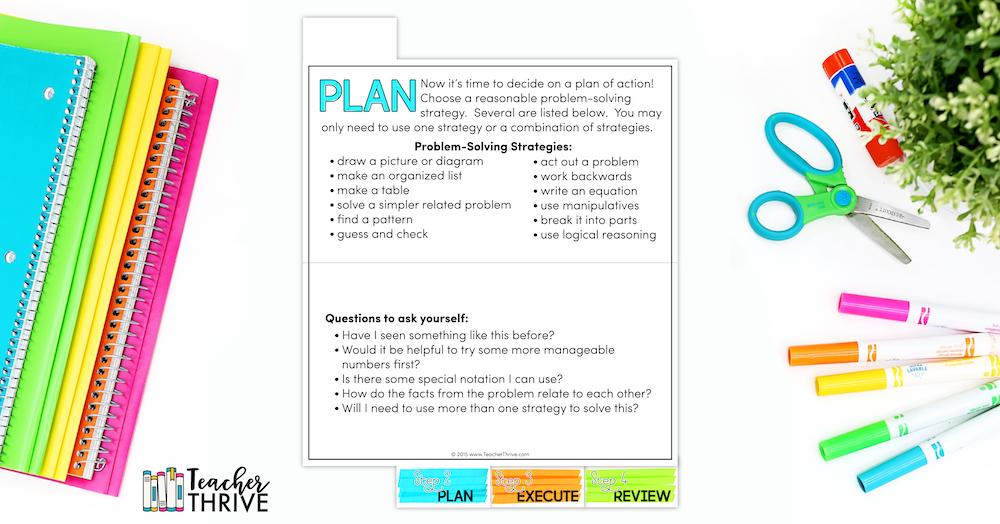Now it’s time to decide on a plan of action! Choose a reasonable problem-solving strategy. Several are listed below.  You may only need to use one strategy or a combination of strategies.

• draw a picture or diagram
• make an organized list
• make a table
• solve a simpler related problem
• find a pattern
• guess and check
• act out a problem
• work backward
• write an equation
• use manipulatives
• break it into parts
• use logical reasoning

## 3. Execute:Alright! You understand the problem.  You have a plan to solve the problem.  Now it’s time to dig in and get to work! As you work, you may need to revise your plan. That’s okay! Your plan is not set in stone and can change anytime you see fit.

• Am I checking each step of my plan as I work?
• Am I keeping an accurate record of my work?
• Am I keeping my work organized so that I could explain my thinking to others?
• Am I going in the right direction? Is my plan working?
• Do I need to go back to Step 2 and find a new plan?
• Do I think I have the correct solution? If so, it’s time to move on to the next step!

## 4. Review: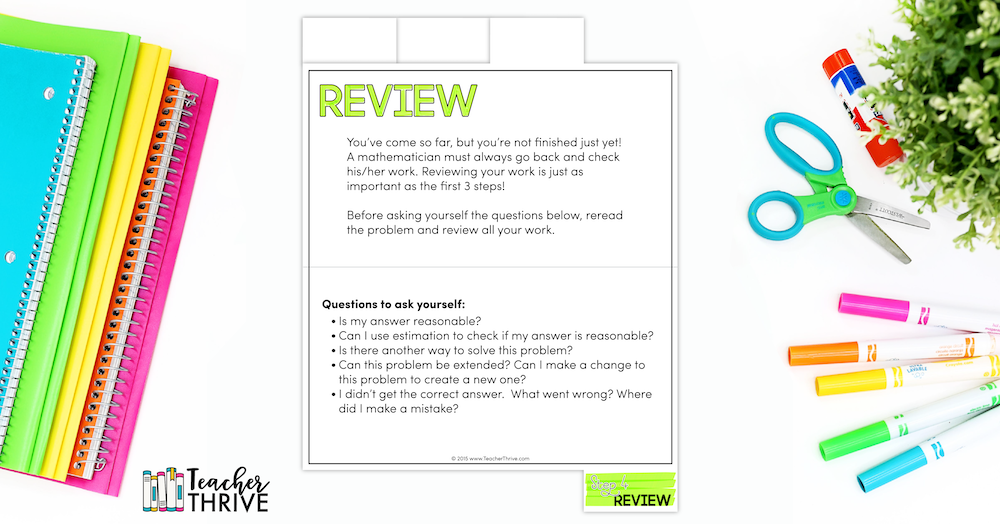You’ve come so far, but you’re not finished just yet!  A mathematician must always go back and check his/her work. Reviewing your work is just as important as the first 3 steps! Before asking yourself the questions below, reread the problem and review all your work.

• Can I use estimation to check if my answer is reasonable?
• Is there another way to solve this problem?
• Can this problem be extended? Can I make a change to this problem to create a new one?
• I didn’t get the correct answer.  What went wrong? Where did I make a mistake?

My Brain Power Math resources are the perfect compliment to this free flip-book.  Each book has a collection of non-routine math problems in a variety of formats.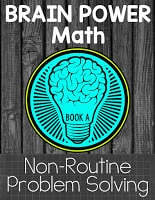## Latest on InstagramMathematical Mysteries

Revealing the mysteries of mathematics

## How to Solve Math Problems: Non-Routine ProblemsNonroutine Problem Solving , stresses the use of heuristics  and often requires little to no use of algorithms. Unlike algorithms, heuristics are procedures or strategies that do not guarantee a solution to a problem but provide a more highly probable method for discovering a solution. Building a model and drawing a picture of a problem are two basic problem-solving heuristics. Studying end-of-game situations provides students with experiences in using the heuristics of reducing the problem to a similar but simpler problem and working a problem backwards, i.e. from its resolution to its initial state. Other heuristics include describing the problem situation, classifying information, and finding irrelevant information. 

## There Are Two Categories of Nonroutine Problem Solving: Static and Active

Static-Nonroutine  problems have a fixed, known goal and fixed, known elements that are used to resolve the problem. Solving a jigsaw puzzle is an example of a Static- Nonroutine problem. Given all pieces to a puzzle and a picture of the goal, learners are challenged to arrange the pieces to complete the picture. Various heuristics such as classifying the pieces by color, connecting the pieces that form the border, or connecting the pieces that form a salient feature to the puzzle, such as a flag pole, are typical ways in which people attempt to resolve such problems. 

Active-Nonroutine  problems may have a fixed goal with changing elements, a changing goal or alternative goals with fixed elements, or changing or alternative goals with changing elements. The heuristics used in this form of problem-solving are known as strategies. People who study such problems must learn to change or adapt their strategies as the problem unfolds.## What is non-routine problem-solving in math?

A non-routine problem is any complex problem that requires some degree of creativity or originality to solve. Non-routine problems typically do not have an immediately apparent strategy for solving them. Often times, these problems can be solved in multiple ways.

Incorporating non-routine problem solving into your math program is one of the most impactful steps you can take as an educator. By consistently allowing your students to grapple with these challenging problems, you are helping them acquire essential problem-solving skills and the confidence needed to successfully execute them. 

## Step 1: Understand

This is a time to just think! Allow yourself some time to get to know the problem. Read and reread. No pencil or paper necessary for this step. Remember, you cannot solve a problem until you know what the problem is!

• Does the problem give me enough information (or too much information)?
• What question is being asked of me?
• What do I know and what do I need to find out?
• What should my solution look like?
• What type of mathematics might be required?
• Can I restate the problem in my own words?
• Are there any terms or words that I am unfamiliar with?

## Step 2: Plan

Now it’s time to decide on a plan of action! Choose a reasonable problem-solving strategy. Several are listed below. You may only need to use one strategy or a combination of strategies.

• Draw a picture or diagram
• Make an organized list
• Make a table
• Solve a simpler related problem
• Find a pattern
• Guess and check
• Act out a problem
• Work backward
• Write an equation
• Use manipulatives
• Break it into parts
• Use logical reasoning

## Step 3: Execute

Alright! You understand the problem. You have a plan to solve the problem. Now it’s time to dig in and get to work! As you work, you may need to revise your plan. That’s okay! Your plan is not set in stone and can change anytime you see fit.

• Am I checking each step of my plan as I work?
• Am I keeping an accurate record of my work?
• Am I keeping my work organized so that I could explain my thinking to others?
• Am I going in the right direction? Is my plan working?
• Do I need to go back to Step 2 and find a new plan?
• Do I think I have the correct solution? If so, it’s time to move on to the next step!

## Step 4: Review

You’ve come so far, but you’re not finished just yet! A mathematician must always go back and check his/her work. Reviewing your work is just as important as the first 3 steps! Before asking yourself the questions below, reread the problem and review all your work.

• Can I use estimation to check if my answer is reasonable?
• Is there another way to solve this problem?
• Can this problem be extended? Can I make a change to this problem to create a new one?
• I didn’t get the correct answer. What went wrong? Where did I make a mistake?

 “Pentathlon Institute Active Problem-Solving”. 2023.  mathpentath.org . https://www.mathpentath.org/active-problem-solving/ .

 Tallman, Melissa. 2015. “Problem Solving In Math • Teacher Thrive”. Teacher Thrive. https://teacherthrive.com/non-routine-problem-solving/ .

 A heuristic is  a mental shortcut commonly used to simplify problems and avoid cognitive overload .

“101 Great Higher-Order Thinking Questions for Math”. 2023.  elementaryassessments.com . https://elementaryassessments.com/higher-order-thinking-questions-for-math/ .

⭐ “Developing Mathematics Thinking with HOTS (Higher Order Thinking Skills) Questions”. 2023. saydel.k12.ia.us . https://www.saydel.k12.ia.us/cms_files/resources/Developing%20Mathematics%20Thinking%20with%20HOTS%20Questions%20(from%20classroom%20observations)PDF.pdf .

“Higher Order Thinking Skills in Maths”. 2017.  education.gov.Scot . https://education.gov.scot/resources/higher-order-thinking-skills-in-maths/ .

“How to Increase Higher Order Thinking”. 2023.  Reading Rockets . https://www.readingrockets.org/topics/comprehension/articles/how-increase-higher-order-thinking .

⭐ I suggest that you read the entire reference. Other references can be read in their entirety but I leave that up to you.• Report this content
• Manage subscriptions
• Collapse this bar#### IMAGES

1. Mathematics Quarter 4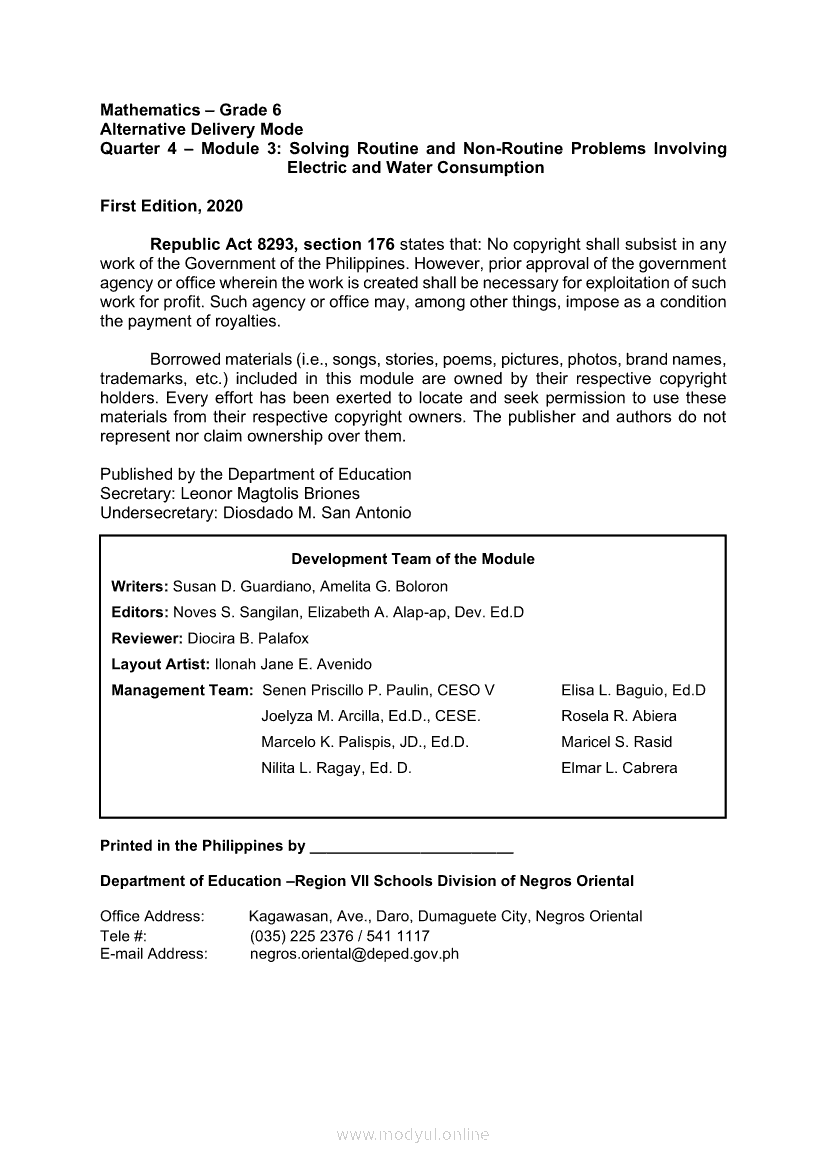2. MATHEMATICS Quarter 4: Module 4 Reading, Measuring and Solving Routine and Non-Routine Problems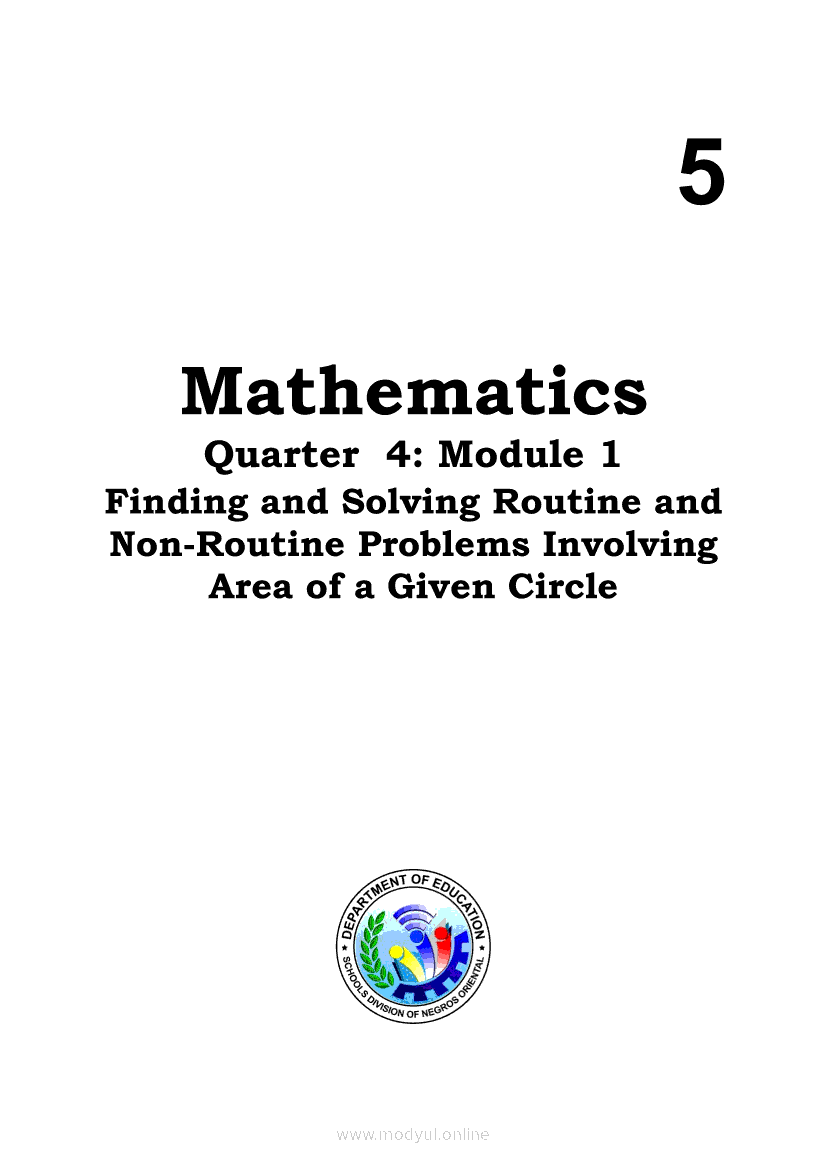3. Non Routine Solving Problem4. Non-Routine Problem Solving in Math5. Image result for non routine problems in mathematics worksheets (With images)6. Image result for non routine problems in mathematics worksheets#### VIDEO

1. Math Problems 😱🤓

2. A Nice Math Problem , (14!-13!)/12!

3. A Nice Math Problem

4. Maths

5. Math4 week8 Q4: Solving Routine andNon-Routine Problems Involving aSimple Experiment| withanswerkey

6. Do 30 math problems in 30 days

The answer to any math problem depends on upon the question being asked. In most math problems, one needs to determine a missing variable. For instance, if a problem reads as 2+3 = , one needs to figure out what the number after the equals ...

2. What Is the Way to Find a Missing Number in a Math Problem?

It depends on the operation being performed within the math problem, but finding a missing number typically requires the student to perform the opposite operation on both sides of the equation.

3. How Do You Do Math Percentage Problems?

To calculate percentages, convert the percentage to a decimal and multiply it by the number in the problem. For example, to find 40 percent of 50, change it to 0.40 times 50, which gives you the result of 20.

4. Non-Routine Mathematics

Creative problem solving or Non-Routine Mathematics involves finding solutions for unseen problems or situations that are different from structured Maths

5. What is non-routine problem-solving in math?

What is non-routine problem-solving in math? A non-routine problem is any complex problem that requires some degree of creativity or originality to solve. Non-

6. What is the difference between routine and non-routine problems

Examples of non-routine problems include creating a new business plan, developing a novel product, or solving a complex Math problem. 1.

7. Non-Routine Problem Solving in Math

Learn how to get your students thinking critically by solving non-routine problems in math. I also share how you can access a free

8. Routine and non-routine problem solving

Doing the arithmetic is secondary to the matter. A mathematics researcher interviewed children about how they solve routine problems. One boy reported his

9. Difficulties in Solving Non-Routine Problems

Although many students struggle with problem-solving. (Garderen, 2006; Tarzimah & Thamby Subahan, 2010), they still need to learn mathematics especially algebra

10. Learning To Solve Non-routine Mathematical Problems

Key Words: Problem solving, problem solving strategies, non-routine problems, mathematics teaching. INTRODUCTION. Many research studies and projects have

11. Ten Non-Routine Problem Solving Favorites for Math 129

Ten Non-Routine Problem Solving Favorites for Math 129. Compiled by Oakley D (O.D.) Hadfield. New Mexico State University. Problem 1 - Paint Mixing. A painter

12. non-routine problems in primary mathematics

In one of the studied textbook half of the problems are word problems, in the other one only one quarter. Keywords: mathematical problem solving; non-routine

13. How to Solve Math Problems: Non-Routine Problems

Nonroutine Problem Solving, stresses the use of heuristics  and often requires little to no use of algorithms. Unlike algorithms, heuristics are

14. Research Article Non-routine problem solving and strategy flexibility

Leading math educators argue that non-routine problems are indispensable for the development of students' problem-solving and reasoning skills (